Function Repository Resource:

# KochCurvePoints

Compute the points that form a generalized Koch curve

Contributed by: Garrett Dubofsky, Wolfram|Alpha Math Team
 ResourceFunction["KochCurvePoints"][n,{p1,p2}] returns a list of points for the nth-step Koch curve with endpoints p1 and p2. ResourceFunction["KochCurvePoints"][n,{p1,p2,…}] joins multiple Koch curves together with endpoints at each successive pair of points. ResourceFunction["KochCurvePoints"][n,{p1,p2,…},orient] determine the direction of the Koch curve with orient. ResourceFunction["KochCurvePoints"][n,m] returns the nth-step Koch snowflake originating from a regular polygon with m sides.

## Details

The Koch curve is interchangable with the Koch snowflake.
The points of the list {p1,p2,} can be either be in 2D or 3D.
The value of orient can be "Up" or "Down" in 2D or any numeric value in 3D.

## Examples

### Basic Examples (2)

The points forming a 1st-step Koch curve:

 In:=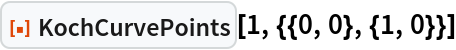Out=A 1st-step Koch curve visualization:

 In:=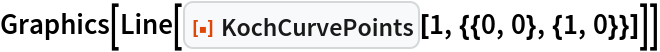Out=Visualizations of the 2nd through 5th-step Koch curves:

 In:=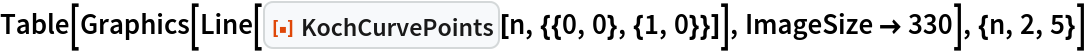Out=A 2nd-step Koch curve in 3 dimensions:

 In:=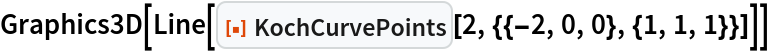Out=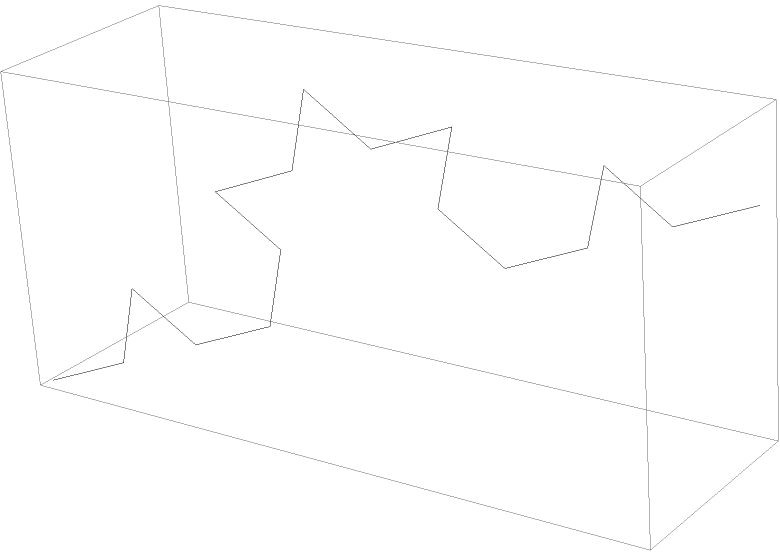### Scope (5)

Form Koch curves over a 2D list of points:

 In:=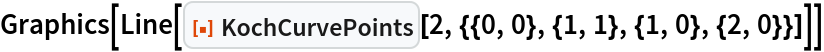Out=Form Koch curves over a 3D list of points:

 In:=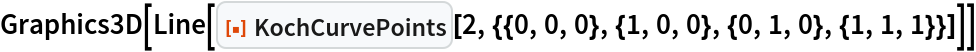Out=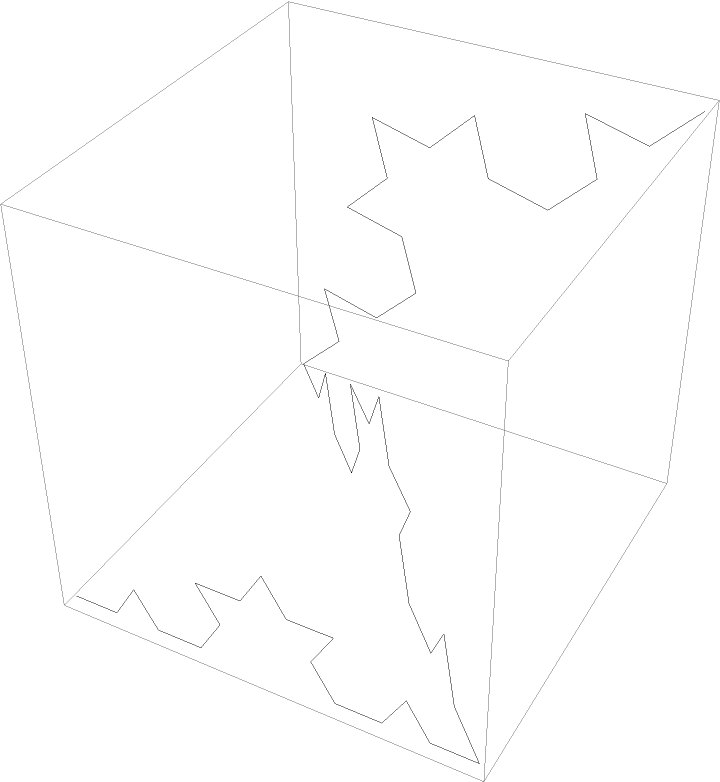Change the path direction of the 2D Koch curve:

 In:=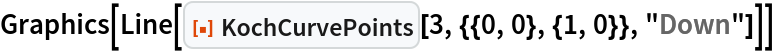Out=Change the path angle of the 3D Koch curve:

 In:=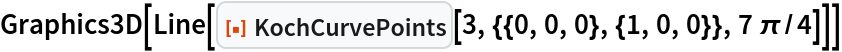Out=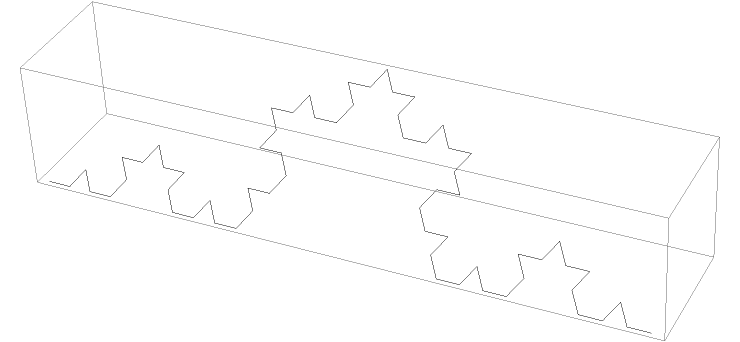A representation of how the angle rotates the 3D Koch curve, where the 0 angle is in black:

 In:=Out=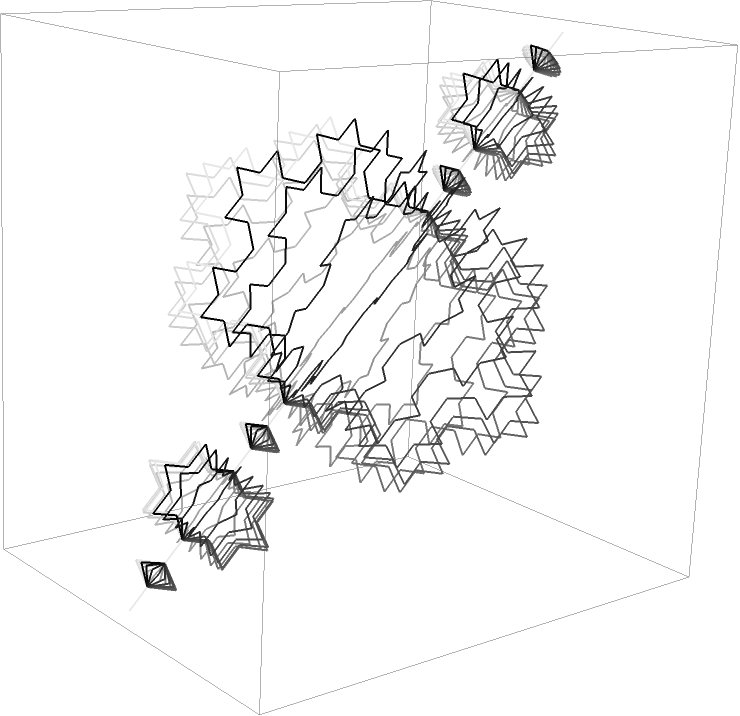The 0th-step Koch Curve does not introduce any new points:

 In:=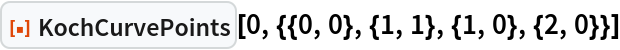Out=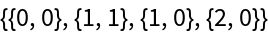### Options (2)

Setting "ExactValues" to False causes the output to be imprecise:

 In:=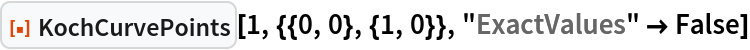Out=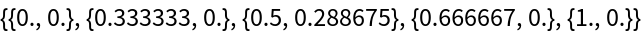Imprecise outputs are much faster to compute:

 In:=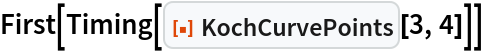Out=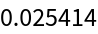In:=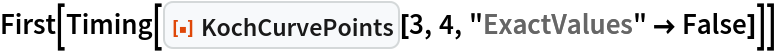Out=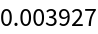### Applications (2)

Form a Koch snowflake by specifying the corner points:

 In:=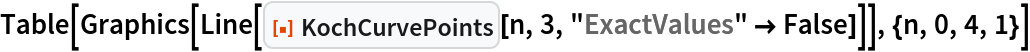Out=Invert the Koch snowflake:

 In:=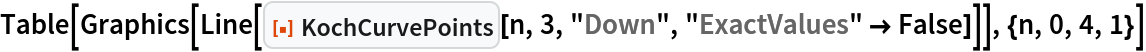Out=A matrix of the first six steps of Koch snowflakes from the first six regular polygons:

 In:=Out=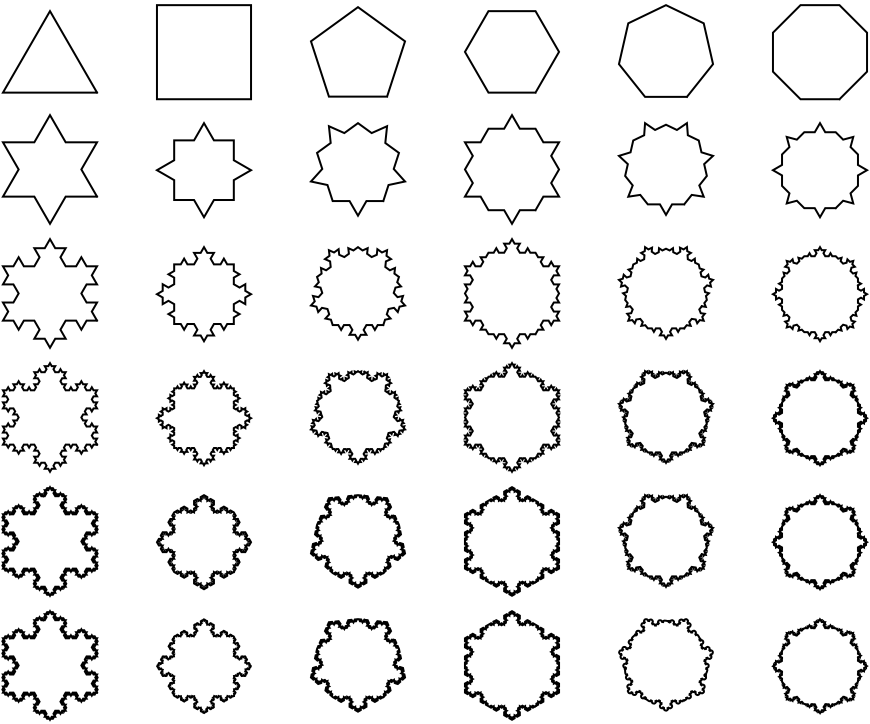### Properties and Relations (1)

The Koch snowflake can be generated with either KochCurve or KochCurvePoints:

 In:=Out=In:=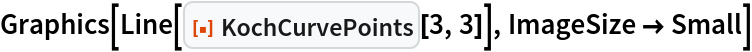Out=### Possible Issues (1)

If a point or the value of the orientation is inexact, then parts of the output will return inexact:

 In:=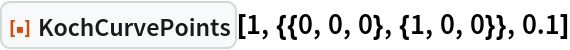Out=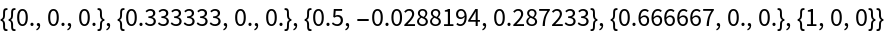In:=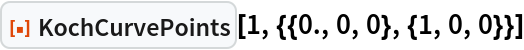Out=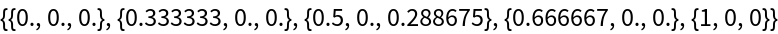### Neat Examples (4)

Increasing the density of lines gives the impression of a solid object:

 In:=Out=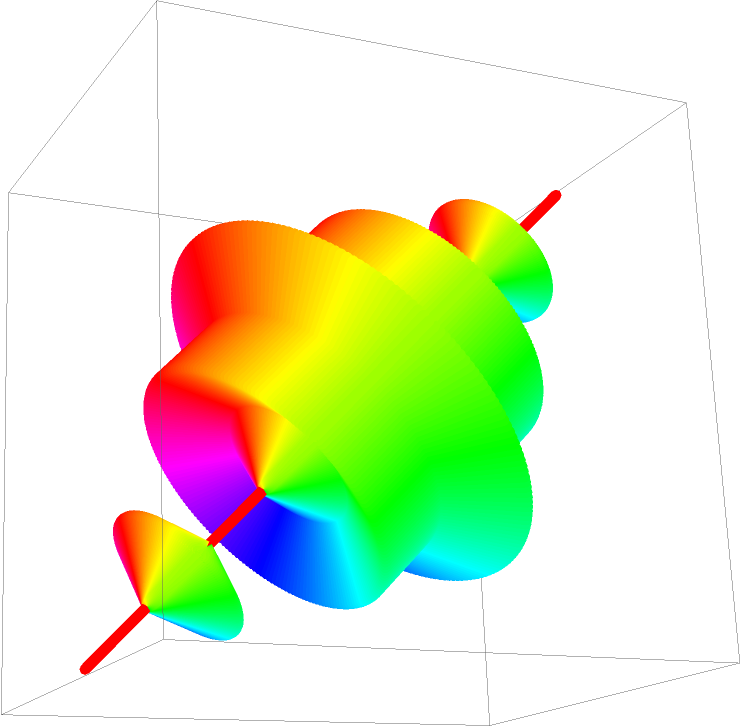Visualize the increasing detail of higher-step Koch curves:

 In:=Out=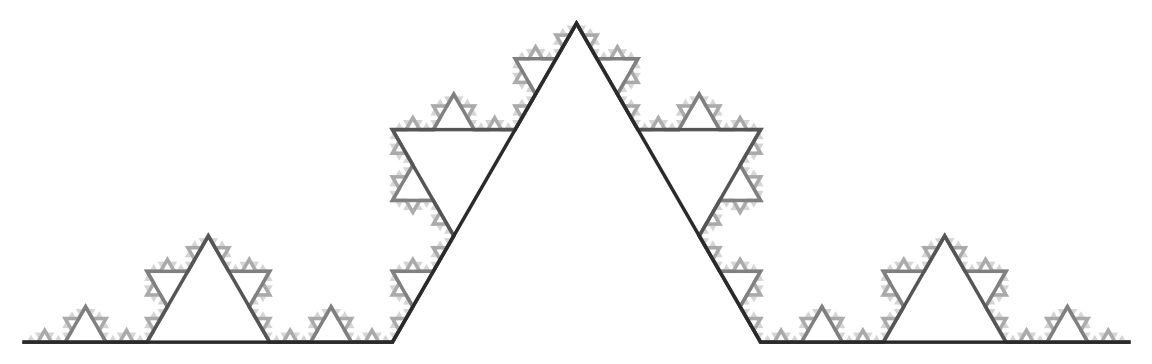Apply the Koch Curve to other named curves:

 In:=Out=An overlap of the Koch Snowflakes generated from the first 10 regular polygons:

 In:=Out=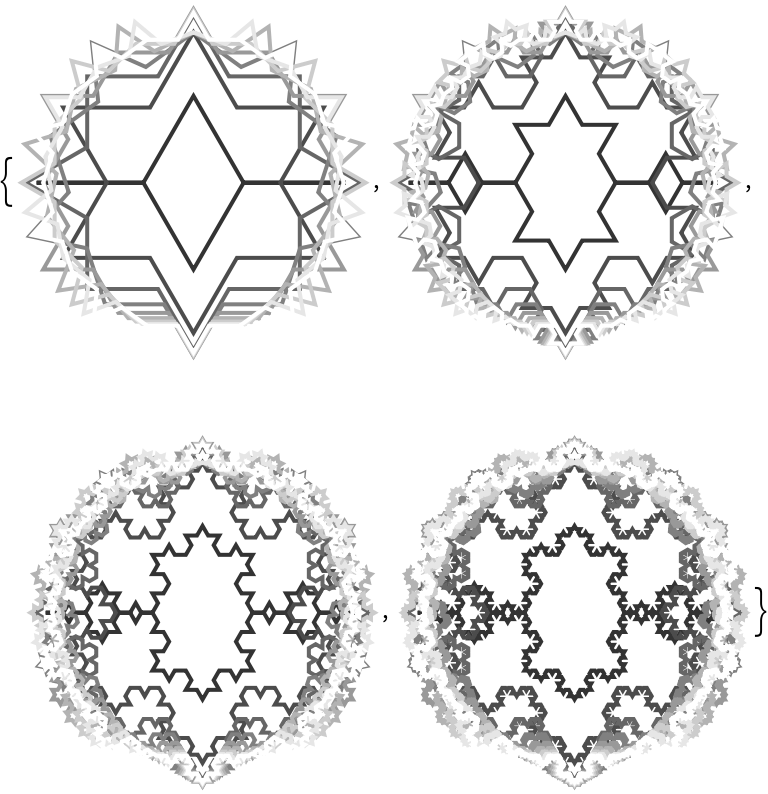The same Koch Snowflakes when the orientation is reversed:

 In:=Out=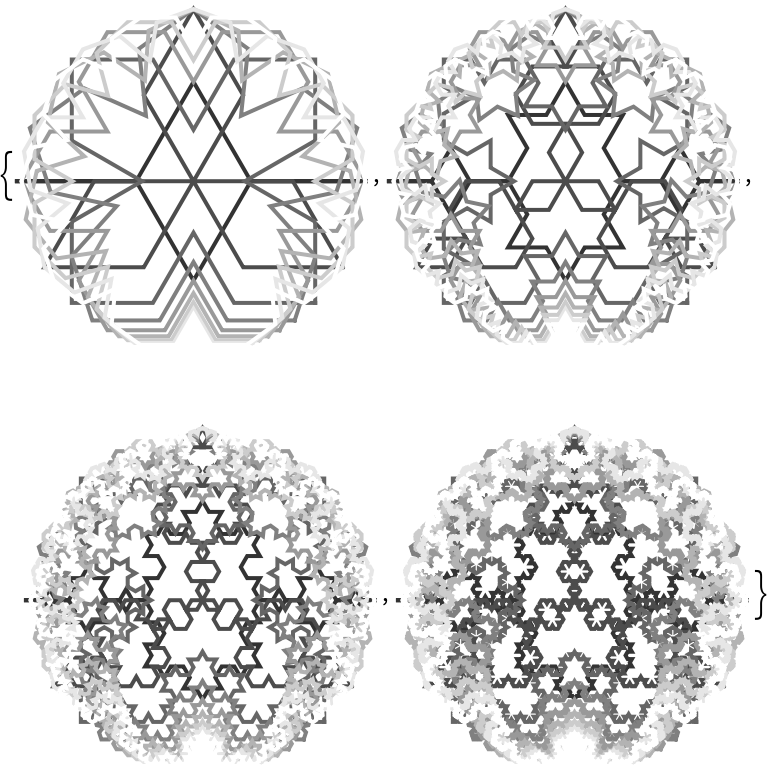## Publisher

Wolfram|Alpha Math Team

## Version History

• 2.0.0 – 23 March 2023
• 1.0.0 – 30 August 2022

## Author Notes

To view the full source code for PolarCurveIdentifier, evaluate the following: